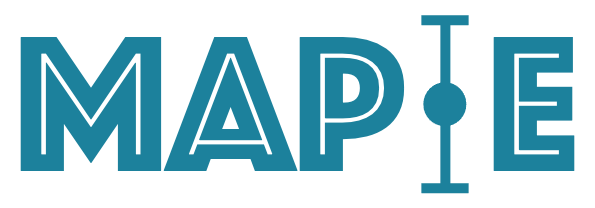A scikit-learn-compatible module for estimating prediction intervals.

## Project description## MAPIE - Model Agnostic Prediction Interval Estimator

MAPIE is an open-source Python library for quantifying uncertainties and controlling the risks of machine learning models. It is a scikit-learn-contrib project that allows you to:

• Easily compute conformal prediction intervals (or prediction sets) with controlled (or guaranteed) marginal coverage rate for regression [3,4,8], classification (binary and multi-class) [5-7] and time series .

• Easily control risks of more complex tasks such as multi-label classification, semantic segmentation in computer vision (probabilistic guarantees on recall, precision, …) [10-12].

• Easily wrap any model (scikit-learn, tensorflow, pytorch, …) with, if needed, a scikit-learn-compatible wrapper for the purposes just mentioned.

Here’s a quick instantiation of MAPIE models for regression and classification problems related to uncertainty quantification (more details in the Quickstart section):

# Uncertainty quantification for regression problem
from mapie.regression import MapieRegressor
mapie_regressor = MapieRegressor(estimator=regressor, method='plus', cv=5)
# Uncertainty quantification for classification problem
from mapie.classification import MapieClassifier
mapie_classifier = MapieClassifier(estimator=classifier, method='score', cv=5)

Implemented methods in MAPIE respect three fundamental pillars:

• They are model and use case agnostic,

• They possess theoretical guarantees under minimal assumptions on the data and the model,

• They are based on peer-reviewed algorithms and respect programming standards.

MAPIE relies notably on the field of Conformal Prediction and Distribution-Free Inference.

## 🔗 Requirements

• MAPIE runs on Python 3.7+.

• MAPIE stands on the shoulders of giants. Its only internal dependencies are scikit-learn and numpy=>1.21.

## 🛠 Installation

MAPIE can be installed in different ways:

$pip install mapie # installation via pip$ conda install -c conda-forge mapie  # or via conda
\$ pip install git+https://github.com/scikit-learn-contrib/MAPIE  # or directly from the github repository

## ⚡ Quickstart

Here we propose two basic uncertainty quantification problems for regression and classification tasks with scikit-learn.

As MAPIE is compatible with the standard scikit-learn API, you can see that with just these few lines of code:

• How easy it is to wrap your favorite scikit-learn-compatible model around your model.

• How easy it is to follow the standard sequential fit and predict process like any scikit-learn estimator.

# Uncertainty quantification for regression problem
import numpy as np
from sklearn.linear_model import LinearRegression
from sklearn.datasets import make_regression
from sklearn.model_selection import train_test_split

from mapie.regression import MapieRegressor

X, y = make_regression(n_samples=500, n_features=1)
X_train, X_test, y_train, y_test = train_test_split(X, y, test_size=0.5)

regressor = LinearRegression()

mapie_regressor = MapieRegressor(estimator=regressor, method='plus', cv=5)

mapie_regressor = mapie_regressor.fit(X_train, y_train)
y_pred, y_pis = mapie_regressor.predict(X_test, alpha=[0.05, 0.32])
# Uncertainty quantification for classification problem
import numpy as np
from sklearn.linear_model import LogisticRegression
from sklearn.datasets import make_blobs
from sklearn.model_selection import train_test_split

from mapie.classification import MapieClassifier

X, y = make_blobs(n_samples=500, n_features=2, centers=3)
X_train, X_test, y_train, y_test = train_test_split(X, y, test_size=0.5)

classifier = LogisticRegression()

mapie_classifier = MapieClassifier(estimator=classifier, method='score', cv=5)

mapie_classifier = mapie_classifier.fit(X_train, y_train)
y_pred, y_pis = mapie_classifier.predict(X_test, alpha=[0.05, 0.32])

## 📘 Documentation

The full documentation can be found on this link.

## 📝 Contributing

You are welcome to propose and contribute new ideas. We encourage you to open an issue so that we can align on the work to be done. It is generally a good idea to have a quick discussion before opening a pull request that is potentially out-of-scope. For more information on the contribution process, please go here.

## 🤝 Affiliations

MAPIE has been developed through a collaboration between Quantmetry, Michelin, ENS Paris-Saclay, and with the financial support from Région Ile de France and Confiance.ai.

MAPIE is free and open-source software licensed under the 3-clause BSD license.

## Project details

This version0.7.00.6.50.6.40.6.30.6.20.6.10.6.00.5.00.4.20.4.10.4.00.3.20.3.10.3.00.2.30.2.20.2.10.2.00.1.40.1.30.1.2

Uploaded source
Uploaded py3